# LINQ Distinct Method in C#

## LINQ Distinct Method in C# with Examples

In this article, I am going to discuss the LINQ Distinct Method in C# using Examples. Please read our previous article where we discussed the basics of LINQ Set Operators. At the end of this article, you will understand the following pointers.

1. What is LINQ Distinct Method in C#?
2. Examples of LINQ Distinct Method using both Method and Query Syntax
3. How to implement IEqualityComparer?
##### What is LINQ Distinct Method in C#?

The LINQ Distinct Method in C# is used to return the distinct elements from a single data source. There are two overloaded versions available for the Distinct Method as shown below.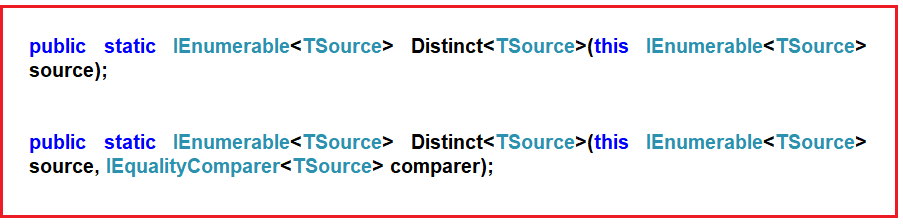The one and only difference between these two methods is the second overloaded version take an IEqualityComparer as an input parameter which means the Distinct Method can also be used with Comparer also. If this is not clear at the moment, don’t worry we will cover the use of the Comparer in this article also.

##### Example to Understand LINQ Distinct Method on Value Type using C#

Here we have an integer collection that contains duplicate integer values. Our requirement is to remove the duplicate values and return only the distinct values as shown below.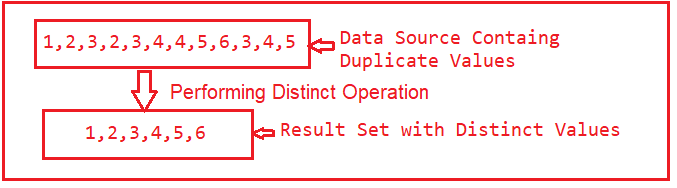The following example shows how to get the distinct integer values from the data source using both Method and Mixed syntax using LINQ Distinct Extension Method. In Query Syntax, there is no such operator call distinct, so we need to use both Query and Method syntax to achieve the same.

```using System;
using System.Collections.Generic;
using System.Linq;

namespace LINQDemo
{
class Program
{
static void Main(string[] args)
{
List<int> intCollection = new List<int>()
{
1,2,3,2,3,4,4,5,6,3,4,5
};

//Using Method Syntax
var MS = intCollection.Distinct();

//Using Query Syntax
var QS = (from num in intCollection
select num).Distinct();
foreach (var item in MS)
{
Console.WriteLine(item);
}

}
}
}```
###### Output: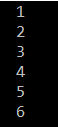##### Example to Understand LINQ Distinct Method with String Values:

Let us see how we can use the LINQ Distinct Method with string values. In the below example, we have a string array of names and we need to return the distinct names from that array collection. To do so, we are using the LINQ Distinct Method.

```using System;
using System.Linq;

namespace LINQDemo
{
class Program
{
static void Main(string[] args)
{
string[] namesArray = { "Priyanka", "HINA", "hina", "Anurag", "Anurag", "ABC", "abc" };

var distinctNames = namesArray.Distinct();

foreach (var name in distinctNames)
{
Console.WriteLine(name);
}

}
}
}
```

When we execute the above program, it gives us the below output.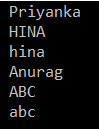As you can see the name Hina and Abc have appeared twice. This is because the default comparer, which is used by the LINQ Distinct method to filter the duplicate values is case-sensitive. So, if you want to make the comparison to be case-insensitive then you need to use the other overloaded version of the Distinct Method which takes IEqualityComparer as an argument. So here we need to pass a class that must implement the IEqualityComparer interface.

So let’s modify the Program class as follows. Here, you can see, we are passing StringComparer as an argument to the LINQ Distinct method and saying OrdinalIgnoreCase which means please ignore the case sensitive while checking the duplicity.

```using System;
using System.Linq;

namespace LINQDemo
{
class Program
{
static void Main(string[] args)
{
string[] namesArray = { "Priyanka", "HINA", "hina", "Anurag", "Anurag", "ABC", "abc" };

var distinctNames = namesArray.Distinct(StringComparer.OrdinalIgnoreCase);

foreach (var name in distinctNames)
{
Console.WriteLine(name);
}

}
}
}
```

With the above changes in place, now run the application and it should display the distinct names as shown in the below image.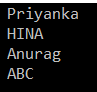Now, if we go to the definition of StringComparer class, then you can see that this class implements the IEqualityComparer interface as shown below. And this is the reason why we can pass this class as a parameter to the Distinct Method.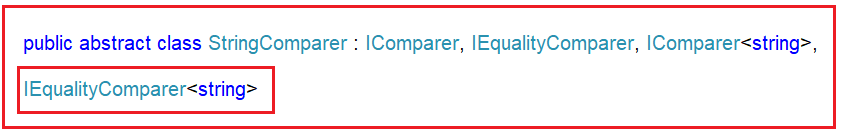##### LINQ Distinct Operation with Complex Data Type using C#:

The LINQ Distinct Method in C# will work in a different manner with complex data types like Employee, Product, Student, etc. Let us understand this with an example. Create a class file with the name Student.cs and then copy and paste the following code into it.

```using System.Collections.Generic;
namespace LINQDemo
{
public class Student
{
public int ID { get; set; }
public string Name { get; set; }

public static List<Student> GetStudents()
{
List<Student> students = new List<Student>()
{
new Student {ID = 101, Name = "Preety" },
new Student {ID = 102, Name = "Sambit" },
new Student {ID = 103, Name = "Hina"},
new Student {ID = 104, Name = "Anurag"},
new Student {ID = 102, Name = "Sambit"},
new Student {ID = 103, Name = "Hina"},
new Student {ID = 101, Name = "Preety" },
};

return students;
}
}
}
```

Here we created the student class with the two properties i.e. ID and Name. Along the same way, we have also created the GetStudents() method which will return a hard-coded collection of students. So, basically, it is returning the following Student data.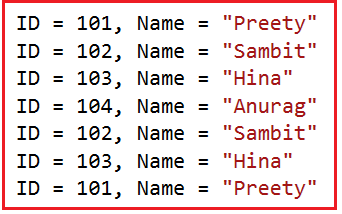##### Example to Understand LINQ Distinct Method with Complex Type in C#:

Let us Understand LINQ Distinct Method with Complex Type in C# with an example. Now, our requirement is to fetch all the distinct names from the student’s collection. The following example shows how to use the LINQ Distinct Method to achieve the same using both Method and Query Syntax.

```using System;
using System.Linq;

namespace LINQDemo
{
class Program
{
static void Main(string[] args)
{
//Using Method Syntax
var MS = Student.GetStudents()
.Select(std => std.Name)
.Distinct().ToList();

//Using Query Syntax
var QS = (from std in Student.GetStudents()
select std.Name)
.Distinct().ToList();

foreach(var item in MS)
{
Console.WriteLine(item);
}

}
}
}```
###### Output: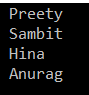In our previous example, we try to retrieve the distinct student names and it works as expected. Now, our requirement is to select distinct students (both ID and Name) from the collection. As you can see in our collection three students are identical and in our result set, they should appear only once. Let us modify the program class as shown below to fetch the distinct student using the LINQ Distinct Method.

```using System;
using System.Linq;

namespace LINQDemo
{
class Program
{
static void Main(string[] args)
{
//Using Method Syntax
var MS = Student.GetStudents()
.Distinct().ToList();

//Using Query Syntax
var QS = (from std in Student.GetStudents()
select std)
.Distinct().ToList();

foreach (var item in QS)
{
Console.WriteLine(\$"ID : {item.ID} , Name : {item.Name} ");
}

}
}
}
```

Now execute the query and see the output.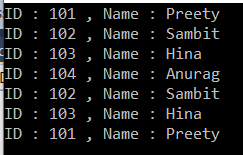As you can see, it will not select distinct students rather it select all the students. This is because the default comparer which is used for comparison by LINQ Distinct Method is only checked whether two object references are equal or not and not the individual property values of the complex object.

##### How to Solve the Above Problem?

We can solve the above problem in four different ways. They are as follows

1. We need to use the other overloaded version of the Distinct() method which takes the IEqualityComparer interface as an argument. So, here we need to create a class that implements the IEqualityComparer interface and then we need to pass that compare instance to the Distinct() method.
2. In the second approach, we need to override the Equals() and GetHashCode() methods within the Student class itself.
3. In the third approach, we need to project the required properties into a new anonymous type, which already overrides the Equals() and GetHashCode() methods
4. By Implementing IEquatable<T> interface.
##### Approach1: Implementing IEqualityComparer Interface

So, create a class file with the name StudentComparer.cs and then implement the IEqualityComparer interface and provide the implementation for Equals and GetHashCode Methods as shown in the below code. Here, within the Equals Method, we are comparing the properties values and if the properties values are same, then we need to return true else false. Also, before accessing the values from the object, we need to make sure that the object itself is not null. Within the GetHashCode Method, we are checking the hash value of the Student Object. And whenever we are implementing the Equals Method, we also need to implement the GetHashCode.

```using System.Collections.Generic;

namespace LINQDemo
{
public class StudentComparer : IEqualityComparer<Student>
{
public bool Equals(Student x, Student y)
{
//First check if both object reference are equal then return true
if(object.ReferenceEquals(x, y))
{
return true;
}

//If either one of the object refernce is null, return false
if (object.ReferenceEquals(x,null) || object.ReferenceEquals(y, null))
{
return false;
}

//Comparing all the properties one by one
return x.ID == y.ID && x.Name == y.Name;
}

public int GetHashCode(Student obj)
{
//If obj is null then return 0
if (obj == null)
{
return 0;
}

//Get the ID hash code value
int IDHashCode = obj.ID.GetHashCode();

//Get the string HashCode Value
//Check for null refernece exception
int NameHashCode = obj.Name == null ? 0 : obj.Name.GetHashCode();

return IDHashCode ^ NameHashCode;
}
}
}
```

Now we need to create an instance of StudentComparer class and then we need to pass that instance to the Distinct method. So, modify the Main Method of the Program class as shown in below.

```using System;
using System.Linq;

namespace LINQDemo
{
class Program
{
static void Main(string[] args)
{
//Creating an instance of StudentComparer
StudentComparer studentComparer = new StudentComparer();

//Using Method Syntax
var MS = Student.GetStudents()
.Distinct(studentComparer).ToList();

//Using Query Syntax
var QS = (from std in Student.GetStudents()
select std)
.Distinct(studentComparer).ToList();

foreach (var item in QS)
{
Console.WriteLine(\$"ID : {item.ID} , Name : {item.Name} ");
}

}
}
}```

With the above changes in place, now run the application and it should display the distinct students as expected as shown in the below image.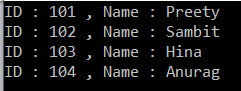##### Approach2: Overriding Equals() and GetHashCode() Methods within the Student Class

As we already know, by default any type in .NET is inherited from the Object class. That means the Student class is also inherited from the Object class. And, we also know that the Object class provides some virtual methods such as Equals() and GetHashCode(). Now, we need to override the Equals() and GetHashCode() methods of the Object class within the Student class. So, modify the Student class as shown below. Here, we are overriding the Equals() and GetHashCode() methods.

```using System.Collections.Generic;
namespace LINQDemo
{
public class Student
{
public int ID { get; set; }
public string Name { get; set; }

public static List<Student> GetStudents()
{
List<Student> students = new List<Student>()
{
new Student {ID = 101, Name = "Preety" },
new Student {ID = 102, Name = "Sambit" },
new Student {ID = 103, Name = "Hina"},
new Student {ID = 104, Name = "Anurag"},
new Student {ID = 102, Name = "Sambit"},
new Student {ID = 103, Name = "Hina"},
new Student {ID = 101, Name = "Preety" },
};

return students;
}

public override bool Equals(object obj)
{
//As the obj parameter type is object, so we need to
//cast it to Student Type
return this.ID == ((Student)obj).ID && this.Name == ((Student)obj).Name;
}

public override int GetHashCode()
{
//Get the ID hash code value
int IDHashCode = this.ID.GetHashCode();

//Get the string HashCode Value
//Check for null refernece exception
int NameHashCode = this.Name == null ? 0 : this.Name.GetHashCode();

return IDHashCode ^ NameHashCode;
}
}
}```

With the above changes in the Student class, now modify the Main method of the Program class as shown below. Now, we don’t need to do anything special with the Distinct Method.

```using System;
using System.Linq;

namespace LINQDemo
{
class Program
{
static void Main(string[] args)
{
//Using Method Syntax
var MS = Student.GetStudents()
.Distinct().ToList();

//Using Query Syntax
var QS = (from std in Student.GetStudents()
select std)
.Distinct().ToList();

foreach (var item in MS)
{
Console.WriteLine(\$"ID : {item.ID} , Name : {item.Name} ");
}

}
}
}```

Now execute the above program and it will display the distinct records as expected as shown in the below image.##### Approach 3: Using Anonymous Type

In this approach, we need to project the properties of the Student class into a new anonymous type and it will work as expected. The reason is the Annonymous Type already overrides the Equals() and GetHashCode() methods of the Object Class. So, modify the Main Method of the Program class as follows. Here, you can see, using the Select Projection Operator and Select Method, we are projecting the output to an anonymous type.

```using System;
using System.Linq;

namespace LINQDemo
{
class Program
{
static void Main(string[] args)
{
//Using Method Syntax
var MS = Student.GetStudents()
.Select(std => new { std.ID, std.Name})
.Distinct().ToList();

//Using Query Syntax
var QS = (from std in Student.GetStudents()
select std)
.Select(std => new { std.ID, std.Name })
.Distinct().ToList();

foreach (var item in MS)
{
Console.WriteLine(\$"ID : {item.ID} , Name : {item.Name} ");
}

}
}
}
```

In the above example, we project the ID and Name properties to IEnumeable<’a> means to anonymous type which already overrides the Equals and GetHashCode method. Now run the application and you will see the output as expected as shown in the below image.##### Approach 4: Implementing IEquatble<T> Interface in Student Class.

In this approach, we need to implement the IEquatble<T> Interface in Student Class and need to implement the Equals Method of the IEquatble<T> Interface and we also need to override the GetHashCode method of the Object class. So, modify the Student class as shown below.

```using System.Collections.Generic;
using System;
namespace LINQDemo
{
public class Student : IEquatable<Student>
{
public int ID { get; set; }
public string Name { get; set; }

public static List<Student> GetStudents()
{
List<Student> students = new List<Student>()
{
new Student {ID = 101, Name = "Preety" },
new Student {ID = 102, Name = "Sambit" },
new Student {ID = 103, Name = "Hina"},
new Student {ID = 104, Name = "Anurag"},
new Student {ID = 102, Name = "Sambit"},
new Student {ID = 103, Name = "Hina"},
new Student {ID = 101, Name = "Preety" },
};

return students;
}

public bool Equals(Student other)
{
if (object.ReferenceEquals(other, null))
{
return false;
}

if (object.ReferenceEquals(this, other))
{
return true;
}

return this.ID.Equals(other.ID) && this.Name.Equals(other.Name);
}

public override int GetHashCode()
{
int IDHashCode = this.ID.GetHashCode();
int NameHashCode = this.Name == null ? 0 : this.Name.GetHashCode();

return IDHashCode ^ NameHashCode;
}
}
}
```

As you can see, here we have done two things. First, we implement the Equals method of the IEquatable interface and then override the GetHashCode method. With the above changes in place, now modify the Main Method of the Program class as shown below.

```using System;
using System.Linq;

namespace LINQDemo
{
class Program
{
static void Main(string[] args)
{
//Using Method Syntax
var MS = Student.GetStudents()
.Distinct().ToList();

//Using Query Syntax
var QS = (from std in Student.GetStudents()
select std)
.Distinct().ToList();

foreach (var item in MS)
{
Console.WriteLine(\$"ID : {item.ID} , Name : {item.Name} ");
}

}
}
}
```

Run the application and you should see the output as expected as shown in the below image.##### Difference Between IEqualityComparer<T> and IEquatable<T> in C#:

The IEqualityComparer<T> is an interface for an object that performs the comparison on two objects of the type T whereas the IEquatable<T> is also an interface for an object of type T so that it can compare itself to another.

In the next article, I am going to discuss the LINQ Except Method using C# with Examples. I hope this article gives you a very good understanding of the Concept of the LINQ Distinct Method in C# with Examples.